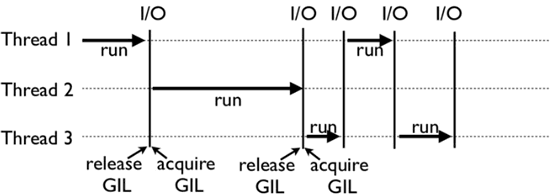# 深度剖析Python GIL全局解释器锁详情

GIL，中文译为全局解释器锁。在讲解 GIL 之前，首先通过一个例子来直观感受一下 GIL 在 Python 多线程程序运行的影响。

```import time
start = time.clock()
def CountDown(n):
while n > 0:
n -= 1
CountDown(100000)
print("Time used:",(time.clock() - start))```

Time used: 0.0039529000000000005

```import time
start = time.clock()
def CountDown(n):
while n > 0:
n -= 1
t1 = Thread(target=CountDown, args=[100000 // 2])
t2 = Thread(target=CountDown, args=[100000 // 2])
t1.start()
t2.start()
t1.join()
t2.join()
print("Time used:",(time.clock() - start))```

Time used: 0.006673

CPython 使用引用计数来管理内容，所有 Python 脚本中创建的实例，都会配备一个引用计数，来记录有多少个指针来指向它。当实例的引用计数的值为 0 时，会自动释放其所占的内存。

>>> import sys
>>> a = [] >>> b = a
>>> sys.getrefcount(a)
3

## Python GIL底层实现原理```for (;;) {
if (--ticker < 0) {
ticker = check_interval;
/* Give another thread a chance */
/* Other threads may run now */
}
bytecode = *next_instr++;
switch (bytecode) {
/* execute the next instruction ... */
}
}```

## Python GIL不能绝对保证线程安全

```import threading
n = 0
def foo():
global n
n += 1
for i in range(100):
t.start()
t.join()
print(n)```

>>> import dis
>>> dis.dis(foo)